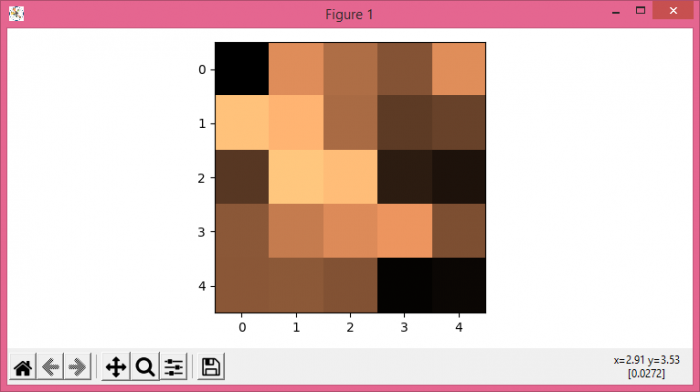# How to save figures to pdf as raster images in Matplotlib?

To save figures to pdf as raster images in Matplotlib, we can take the following steps −

• Set the figure size and adjust the padding between and around the subplots.
• Create a new figure or activate an existing figure.
• Add an axes to the figure as part of a subplot arrangement.
• Create random data using numpy.
• Display the data as an image, i.e., on a 2D regular raster.
• Save the plot as pdf format.

## Example

import numpy as np
import matplotlib.pyplot as plt

plt.rcParams["figure.figsize"] = [7.50, 3.50]
plt.rcParams["figure.autolayout"] = True

fig = plt.figure()
data = np.random.rand(5, 5)

ax.imshow(data, cmap="copper", aspect=True, interpolation="nearest")

plt.savefig("rasterized.pdf")

## Output

When we execute the code, it will save the following plot in the project directory with the name "rasterized.pdf".